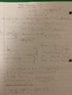# Find the attractive force of gravity between two objects

ac7597
Homework Statement:
Henry Cavendish succeeded in measuring the value of the constant "G" way back in the late 1700s. His method was to put two known masses at a known distance and measure the attractive force between them; then he could use Newton's Law of Universal Gravitation to find "G".
The basic idea was to put a big sphere of a dense material next to a small sphere of dense material. Cavendish chose lead. He made one big ball with radius 5.6 inches, and one small ball with radius 1.9 inches. He then placed them next to each other, with a gap of just 0.5 inches between their closest surfaces.

How large was the attractive force of gravity between these two balls?
Relevant Equations:
G=6.67 * 10 ^(-11)
Homework Statement: Henry Cavendish succeeded in measuring the value of the constant "G" way back in the late 1700s. His method was to put two known masses at a known distance and measure the attractive force between them; then he could use Newton's Law of Universal Gravitation to find "G".
The basic idea was to put a big sphere of a dense material next to a small sphere of dense material. Cavendish chose lead. He made one big ball with radius 5.6 inches, and one small ball with radius 1.9 inches. He then placed them next to each other, with a gap of just 0.5 inches between their closest surfaces.

How large was the attractive force of gravity between these two balls?
G=6.67 * 10 ^(-11)

(mass1)(9.8m/s^2)=(6.67 * 10 ^(-11) ) (mass1)(mass2)/( (5.6in)(0.0254 m) )^2
mass2=2.97 * 10^(9) kg

(mass2)(9.8m/s^2)=(6.67 * 10 ^(-11) ) (mass1)(mass2)/( (1.9in)(0.0254 m) )^2
mass1=342.2 * 10^(6) kg

F=(6.67 * 10 ^(-11) )(342.2 * 10^(6)) (2.97 * 10^(9)) / (0.203m)^2
F=1.645 * 10^(9) N
Apparently this incorrect. I don't know why.

Homework Helper
The problem is your determination of m1 and m2. Why are you using 9.8 m/sec^2? Show us your work in determining these masses.

AM

ac7597
Do I need to find the mass of both objects? Can I somehow cancel them out of the equation?

Homework Helper
Gold Member
2022 Award
Do I need to find the mass of both objects? Can I somehow cancel them out of the equation?

If force depends on the masses, then how could the masses cancel out?

Homework Helper
The density of lead does not appear in your calculations anywhere. You appear to be determining the mass of the balls by assuming that the acceleration of each ball toward the other is 9.8 m/sec^2. That is not correct. You have the mass of a lead ball of radius 1.9 inches at 342 million kilograms!

AM

•phinds
ac7597
This the correct force=1.18 * 10^(-6) N ?

Homework Helper
This the correct force=1.18 * 10^(-6) N ?
You should show us how you arrived at that answer.

AM

ac7597
The top portion is for this question

#### Attachments

•wLKnxWIuQYy%pr5dXFkRdw.jpg
46 KB · Views: 208